# Scale Drawings Scale Factor Ratio A ratio is

• Slides: 24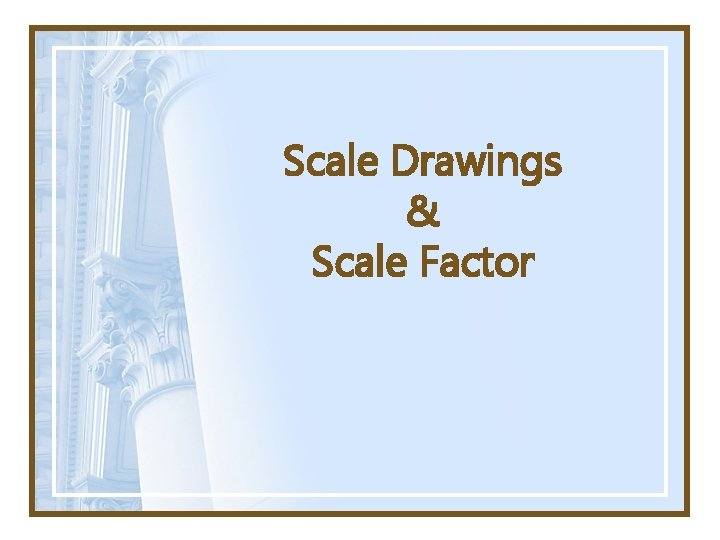Scale Drawings & Scale FactorRatio • A ratio is a comparison of two quantities Proportion • A proportion states that two ratios (or fractions) are equalScale • A scale is a ratio between two sets of measurements. – Examples: • Drawings: ¼ inch = 1 foot • Maps: 1 inch = 250 miles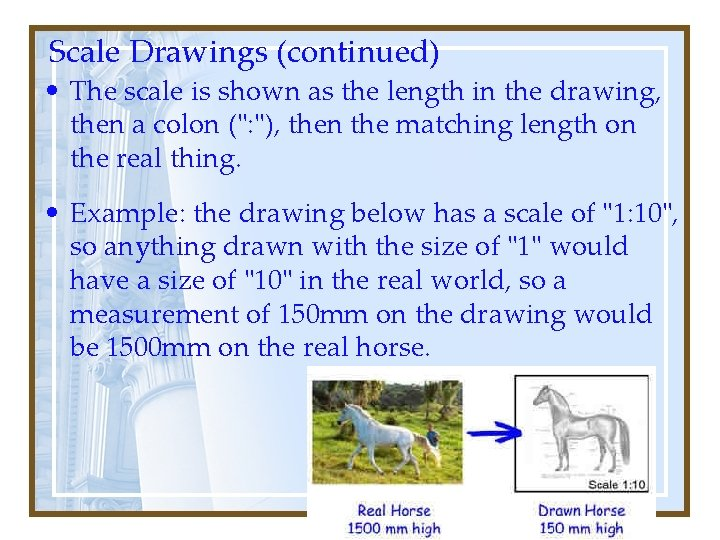Scale Drawings (continued) • The scale is shown as the length in the drawing, then a colon (": "), then the matching length on the real thing. • Example: the drawing below has a scale of "1: 10", so anything drawn with the size of "1" would have a size of "10" in the real world, so a measurement of 150 mm on the drawing would be 1500 mm on the real horse.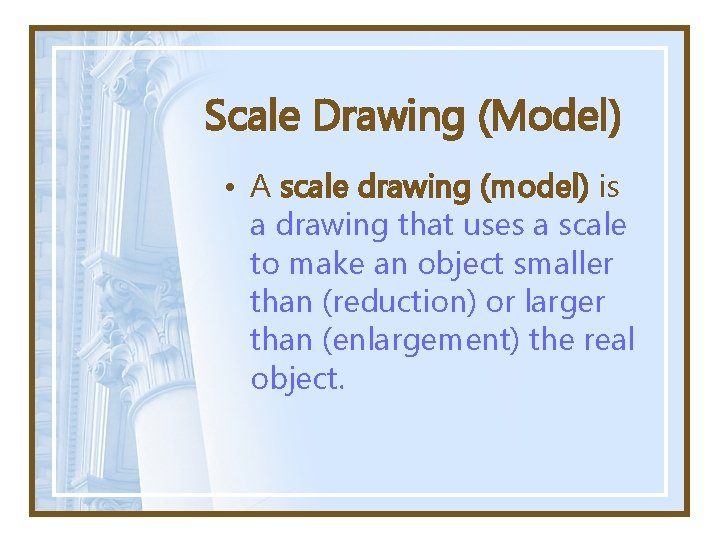Scale Drawing (Model) • A scale drawing (model) is a drawing that uses a scale to make an object smaller than (reduction) or larger than (enlargement) the real object.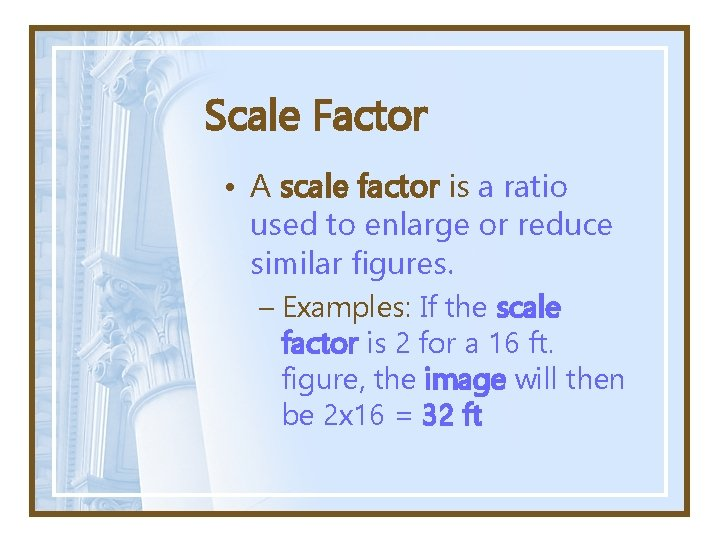Scale Factor • A scale factor is a ratio used to enlarge or reduce similar figures. – Examples: If the scale factor is 2 for a 16 ft. figure, the image will then be 2 x 16 = 32 ft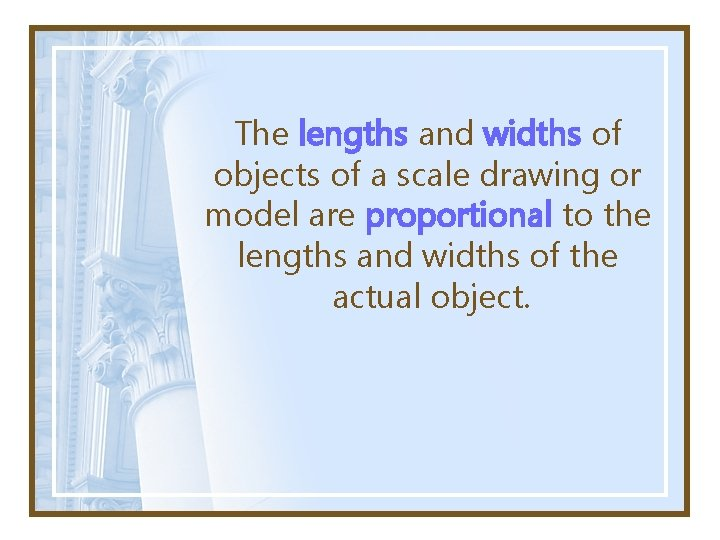The lengths and widths of objects of a scale drawing or model are proportional to the lengths and widths of the actual object.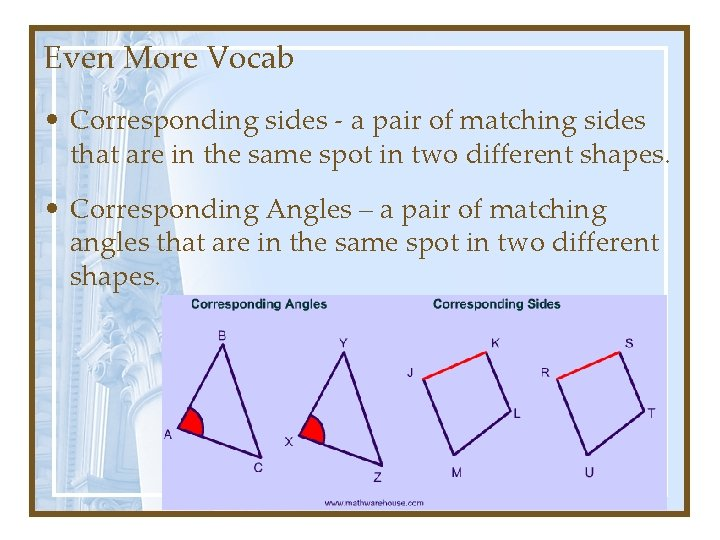Even More Vocab • Corresponding sides - a pair of matching sides that are in the same spot in two different shapes. • Corresponding Angles – a pair of matching angles that are in the same spot in two different shapes.Practice Problems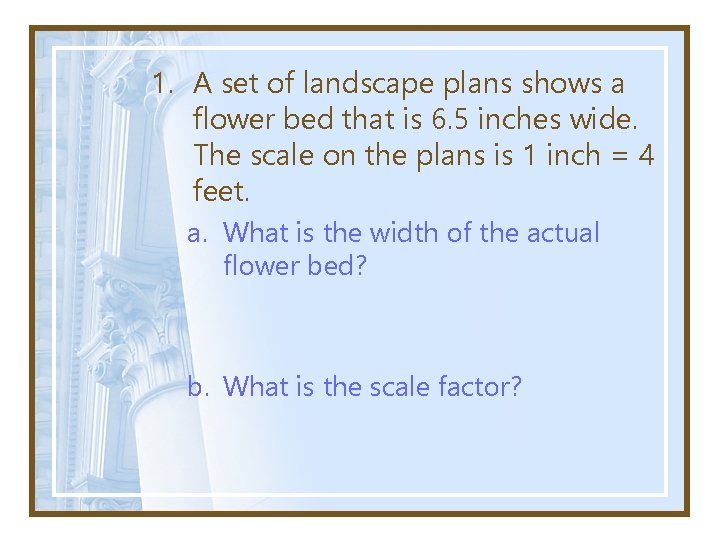1. A set of landscape plans shows a flower bed that is 6. 5 inches wide. The scale on the plans is 1 inch = 4 feet. a. What is the width of the actual flower bed? b. What is the scale factor?2. The central chamber of the Lincoln memorial, which features a marble statue of Abraham Lincoln, has a height of 60 feet. Suppose a scale model of the chamber has a height of 4 inches. What is the scale of the model? a. Write a ratio of the height of the model to the actual height of the statue? b. What is the scale factor?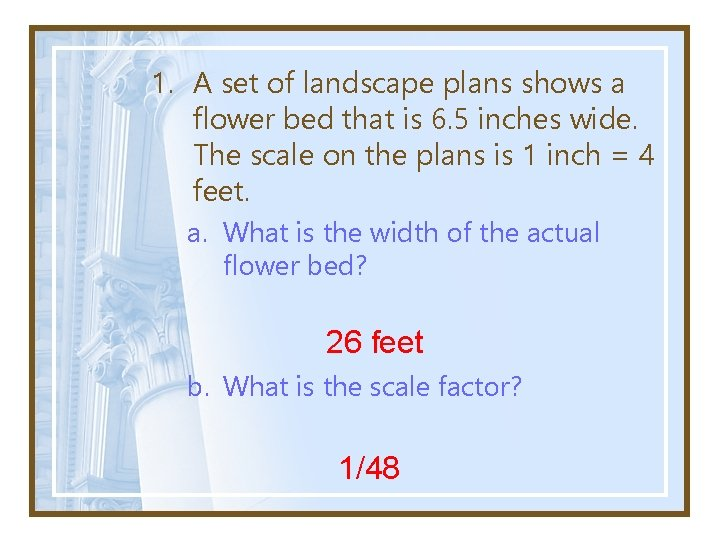1. A set of landscape plans shows a flower bed that is 6. 5 inches wide. The scale on the plans is 1 inch = 4 feet. a. What is the width of the actual flower bed? 26 feet b. What is the scale factor? 1/48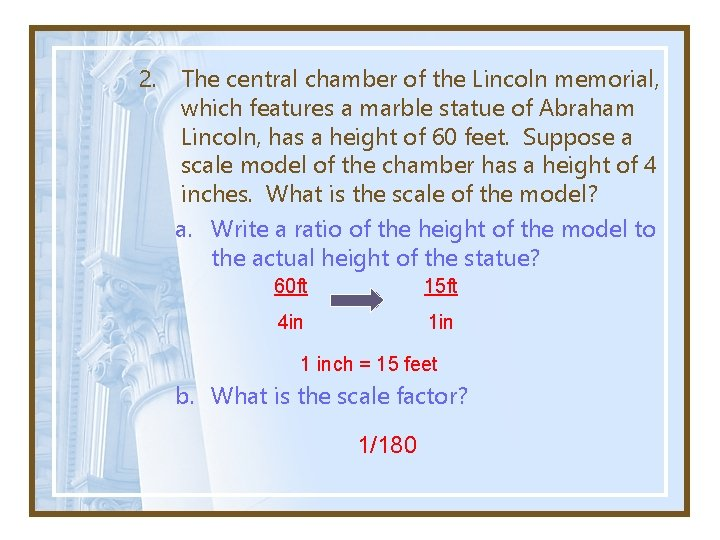2. The central chamber of the Lincoln memorial, which features a marble statue of Abraham Lincoln, has a height of 60 feet. Suppose a scale model of the chamber has a height of 4 inches. What is the scale of the model? a. Write a ratio of the height of the model to the actual height of the statue? 60 ft 15 ft 4 in 1 inch = 15 feet b. What is the scale factor? 1/180The Percent Proportion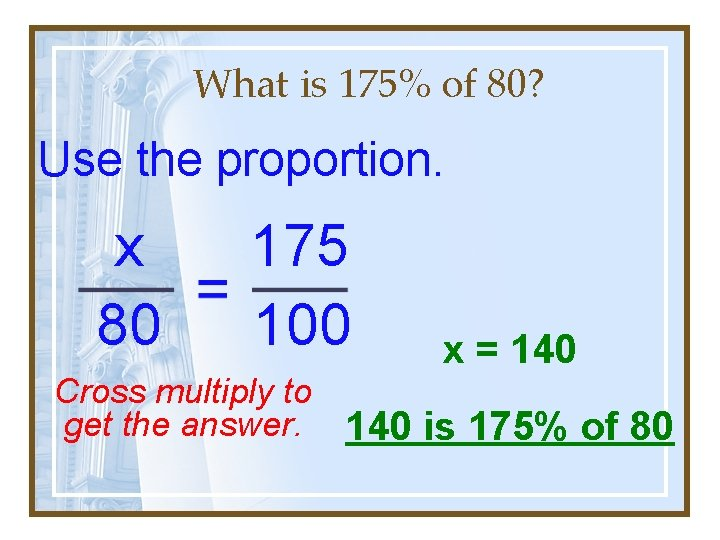What is 175% of 80? Use the proportion. x 80 175 100 x = 140 Cross multiply to get the answer. 140 is 175% of 80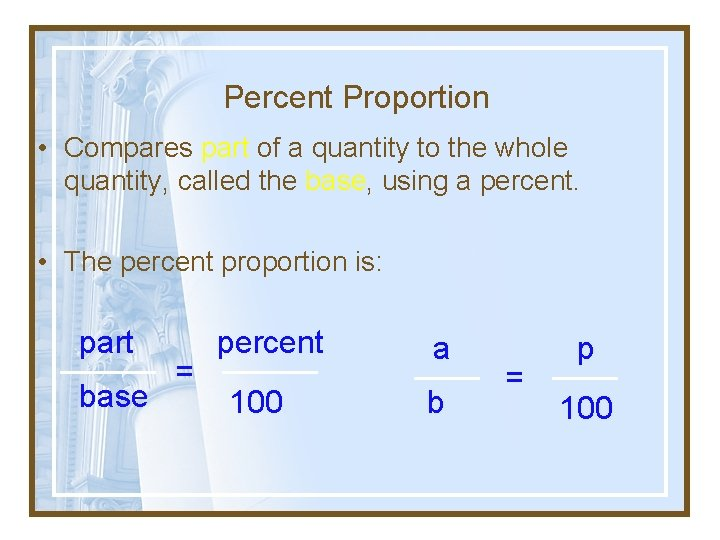Percent Proportion • Compares part of a quantity to the whole quantity, called the base, using a percent. • The percent proportion is: part base = percent 100 a b = p 100Percent Proportion • What percent of \$15 is \$9? The part. The percent is the unknown. The base always follows the word of.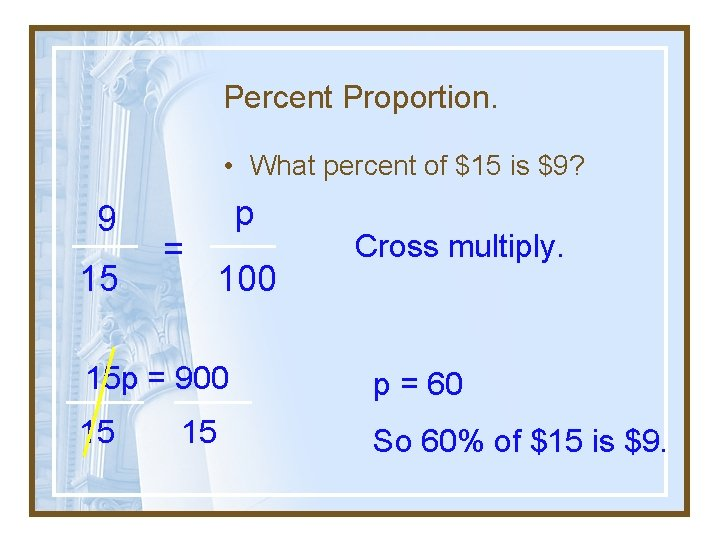Percent Proportion. • What percent of \$15 is \$9? 9 15 = p 100 Cross multiply. 15 p = 900 p = 60 15 So 60% of \$15 is \$9. 15Percent Proportion • What number is 30% of 150? The percent. The part is the unknown. The base always follows the word of.Percent Proportion. • What number is 30% of 150? a 150 = 30 100 a = 4500 100 Cross multiply. a = 45 So 45 is 30% of 150.Percent Proportion • 12 is 80% of what number? The base is the The percent. The part. unknown. The base always follows the word of.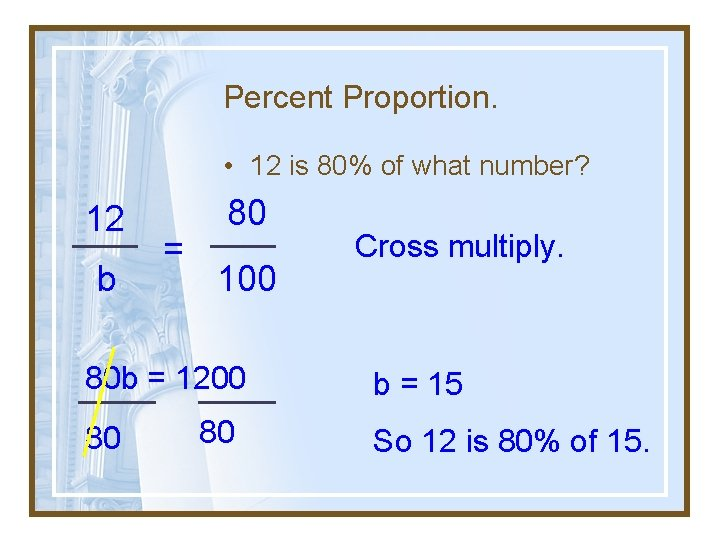Percent Proportion. • 12 is 80% of what number? 12 b = 80 100 80 b = 1200 80 80 Cross multiply. b = 15 So 12 is 80% of 15.Finding Percentages of Enlargement/Reductions • To find the percentage of an enlargement or reduction, we must take two corresponding sides and make a proportion Ex: (do on whiteboard)Homework 66 • • Page 16, #1 -2 Page 18, #6 -11 Page 19 -20, #13 -17, 19 Page 21, #20, 21